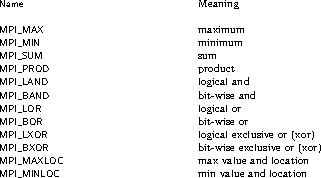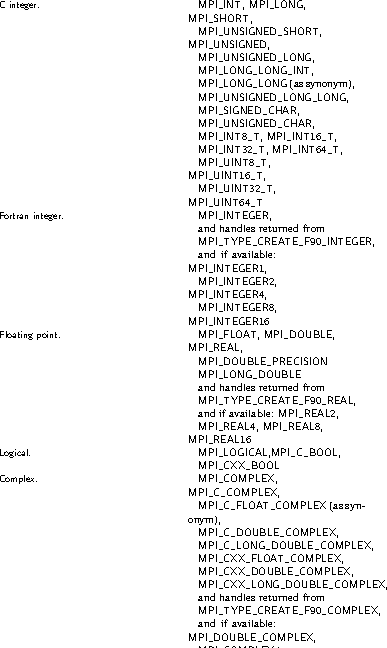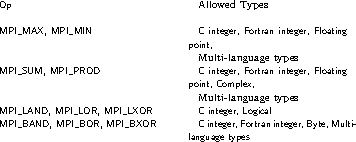## 107. Predefined Reduction OperationsUp: Global Reduction Operations Next: Signed Characters and Reductions Previous: Reduce

The following predefined operations are supplied for MPI_REDUCE and related functions MPI_ALLREDUCE, MPI_REDUCE_SCATTER_BLOCK, MPI_REDUCE_SCATTER, MPI_SCAN, MPI_EXSCAN, all nonblocking variants of those (see Section Nonblocking Collective Operations ), and MPI_REDUCE_LOCAL. These operations are invoked by placing the following in op.The two operations MPI_MINLOC and MPI_MAXLOC are discussed separately in Section MINLOC and MAXLOC . For the other predefined operations, we enumerate below the allowed combinations of op and datatype arguments. First, define groups of MPI basic datatypes in the following way.Now, the valid datatypes for each operation are specified below.These operations together with all listed datatypes are valid in all supported programming languages, see also Reduce Operations in Section MPI Opaque Objects .

The following examples use intracommunicators.

Example A routine that computes the dot product of two vectors that are distributed across a group of processes and returns the answer at node zero.

```SUBROUTINE PAR_BLAS1(m, a, b, c, comm)
REAL a(m), b(m)       ! local slice of array
REAL c                ! result (at node zero)
REAL sum
INTEGER m, comm, i, ierr

! local sum
sum = 0.0
DO i = 1, m
sum = sum + a(i)*b(i)
END DO

! global sum
CALL MPI_REDUCE(sum, c, 1, MPI_REAL, MPI_SUM, 0, comm, ierr)
RETURN
END
```

Example A routine that computes the product of a vector and an array that are distributed across a group of processes and returns the answer at node zero.

```SUBROUTINE PAR_BLAS2(m, n, a, b, c, comm)
REAL a(m), b(m,n)    ! local slice of array
REAL c(n)            ! result
REAL sum(n)
INTEGER n, comm, i, j, ierr

! local sum
DO j= 1, n
sum(j) = 0.0
DO i = 1, m
sum(j) = sum(j) + a(i)*b(i,j)
END DO
END DO

! global sum
CALL MPI_REDUCE(sum, c, n, MPI_REAL, MPI_SUM, 0, comm, ierr)

! return result at node zero (and garbage at the other nodes)
RETURN
END
```Up: Global Reduction Operations Next: Signed Characters and Reductions Previous: Reduce